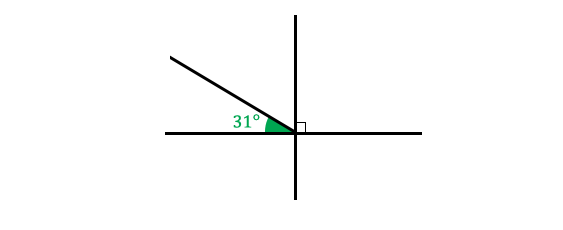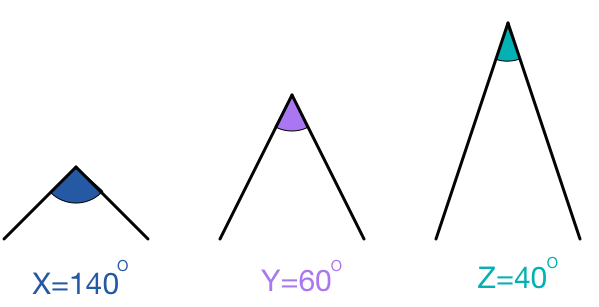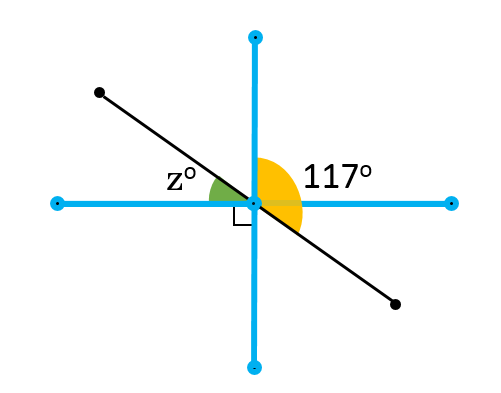Basic Mathematics

# Complementary and Supplementary AnglesGiven that $\color{#20A900}{\text{(green angle)} = 31^\circ},$ which of the following is complementary to that $\color{#20A900}{\text{green angle}}$?Which pair of angles are supplementary?

Suppose $\angle A = 65^\circ$, $\angle B\,$ is complementary to $\angle A,$ and $\angle C$ is supplementary to $\angle B.$ What is the measure of $\angle C ?$

If $\angle A=5 ^\circ$ and $\angle B$ is the supplement of $\angle A,$ then $\angle A + \angle B$ forms a(n) $\text{\_\_\_\_\_\_\_\_\_\_}.$

$\quad \text{A)}$ acute angle
$\quad \text{B)}$ right angle
$\quad \text{C)}$ obtuse angle
$\quad \text{D)}$ straight line
$\quad \text{E)}$ none of the aboveFind the measure of $\angle Z.$

×